# Preprocessor Directives in C++

## Preprocessor Directives in C++ with Examples

In this article, I am going to discuss Preprocessor Directives in C++ which are also called Macros with Examples. Please read our previous article where we discussed Constants in C++ with Examples. As the name suggests, Pre-processors are programs that process our source code before compilation.

##### What are Macros or Preprocessor Directives in C++?

Macros or Preprocessor Directives in C++ are instructions to the compiler. We can give some instructions to the compiler so that before the compiler starts compiling a program, it can follow those instructions and perform those instructions. The most well-known Macros or Pre-Processor Directives that we used in our program is #define.

#define is mostly used for defining some constants. For example,

#define PI 3.1425

Now inside the main function, we can access the PI value as follows:

int main(){
cout << PI;
}

If we have defined PI using #define in our program and the main function is using it, or wherever we are using PI in our program, PI will be replaced by 3.1425. That means wherever PI appears in our program, it will be replaced by 3.1425 there. When will it be replaced? Before the program starts compiling. So, what does the compiler see here in the line cout << PI? The compiler will not see PI, it will see 3.1425. For a better understanding, please have a look at the following.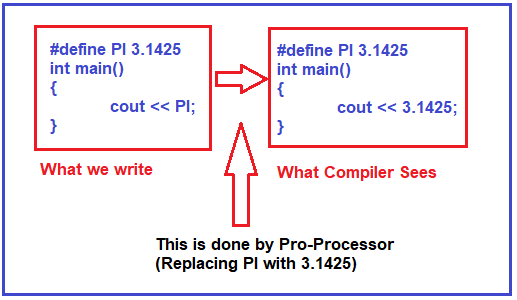The Complete Example Code is given below:

```#include <iostream>
using namespace std;
#define PI 3.1425

int main()
{
cout << PI;
}
```

Output: 3.1425

##### More About #define Pro-Processor Directive in C++:

#define c cout

Now, can we write c << 10? Yes. What will happen? This c will be replaced by cout before the compilation. The compiler will treat this c as cout. So, we can change the object name as well. This is the benefit of the preprocessor. Basically, #define is useful for mentioning some constants. So, these constants are known as symbolic constants. Following is the complete example code.

```#include <iostream>
using namespace std;
#define c cout

int main()
{
c << 10;
}
```

Output: 10

So, by using #define, we can define a symbolic constant.

##### Define Function using #define Preprocessor Directive in C++:

Using #define, we can also define functions. Let us understand this with an example. Please have a look at the below code.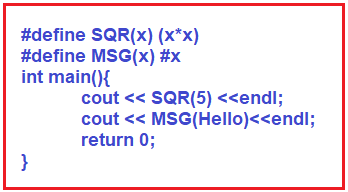Here we have written a function SQR(x) that will perform x*x. Then, within the main function, we wrote cout << SQR(5). So this SQR(5) will be replaced by 5*5. When will it be replaced? It is replaced before the compilation process or precompiler or preprocessor directives. So, it is actually cout << 5*5. It is not SQR for the compiler.

With #x, we created another function, MSG(x). Whatever the parameters we send in MSG, that will be converted into a string. So, if we write cout << MSG(Hello), then it means “Hello”. So, we have given Hello without double-quotes. But MSG (Hello) will be replaced by “Hello”. This is because we have written #x with MSG(x). #x means that the literal or the parameter should be enclosed in double-quotes. So, MSG(Hello) means “Hello” for the compiler. For a better understanding, please have a look at the below image.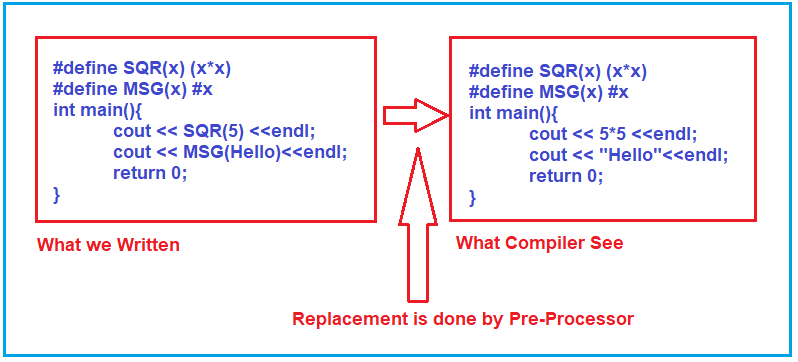The complete example code is given below.

```#include <iostream>
using namespace std;
#define SQR(x) (x*x)
#define MSG(x) #x
int main(){
cout << SQR(5) <<endl;
cout << MSG(Hello)<<endl;
return 0;
}
```
###### Output: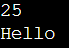This is how we can use directives for writing constants or symbolic constants as well as functions in C++ Language. They are all used to replace the contents before the compilation process starts.

##### #ifndef Directive in C++:

Now let us learn one more thing. We have another keyword that is #ifndef. It means if not defined. If we write,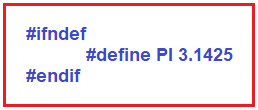This PI is defined if it is not already defined, then only it will be defined, otherwise, it will not be defined again. See, in the program, if the #define is already there, then it will cause an error if you define it again. So that’s why we have written # ifndef, which means if it is not defined, then define it. For a better understanding, please have a look at the following example.

```#include <iostream>
using namespace std;

#define max(x, y) (x > y ? x : y)

#ifndef PI
#define PI 3.1425
#endif

int main()
{
cout << PI << endl;
cout << max (121, 125) << endl;
return 0;
}
```
###### Output: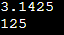##### How a C++ Program Executes?

There are a number of steps involved between writing a C++ Program and Executing that Program. Let us have a look at these steps. Please have a look at the following diagram.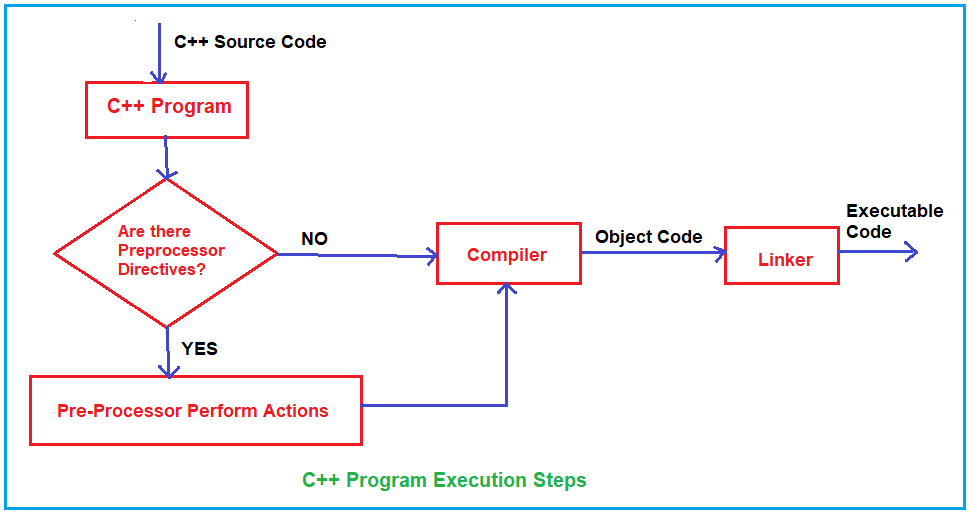As you can see in the above diagram, the source code written by the developer is the first step and the source code is stored in a file with .cpp extension, let us say the file name is MyProgram.cpp. This file is then processed by pre-processors and an expanded source code file is generated with the name MyProgram.i. This expanded file is then compiled by the language compiler and an object code file is generated with the name MyProgram.obj. Finally, the linker links this object code file to the object code of the library functions to generate the executable file MyProgram.exe.

Pre-processor programs provide pre-processor directives that tell the compiler to pre-process the source code before compiling. All of these preprocessor directives begin with a ‘#’ (hash) symbol. The ‘#’ symbol indicates that whatever statement starts with a ‘#’ will go to the pre-processor program to get executed. Examples of some preprocessor directives are: #include, #define, #ifndef etc.